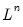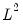Command Reference : Matrix Language Reference

 @cond
Condition number of matrix.
Syntax: @cond(m[, n])
m: matrix, sym
n: (optional) integer
Return: number
Returns the condition number of a square matrix or sym, m.
The condition number is the product of the norm of the matrix divided by the norm of the inverse.
If the norm option n is omitted, the infinity norm is used to determine the condition number. Possible norms are “-1” for the infinity norm, “0” for the Frobenius norm, and an integer “n” for thenorm.
Examples
matrix m1 = @mnrnd(10, 4)
scalar sc1 = @cond(m1)
computes the infinity norm of the matrix M1.
sym s1 = @inner(m1)
scalar sc2 = @cond(s1, 2)
computes thenorm of the symmetric matrix S1.
Cross-references